Trinomial Factoring MathBitsNotebook.com Terms of Use   Contact Person: Donna Roberts"We will be examining quadratic trinomials of the form ax2 + bx + c."Part 1: Trinomials with a = 1 (ax2 + bx + c)

Method by Trial and Error:

Factor: x2 - x - 12
Step 1: The first step when factoring is to always check for a common factor among the terms. In this situation, there is no common factor.
Nope!
Step 2: To get the leading term of x2, each first term of the factors will need to be x.
(x        )(x        )
Step 3: The product of the last terms must be -12. Unfortunately, there are a variety of ways to arrive at this product of -12.
 +12 and -1 -12 and +1 +6 and - 2 -6 and +2 +4 and -3 -4 and +3 ALL of these pairings yield a product of -12. But only ONE of them is the correct choice!
(x + 12)(x - 1)
(x - 12)(x + 1)
(x + 6)(x - 2)
(x - 6)(x + 2)
(x + 4)(x - 3)
(x - 4)(x + 3)

Step 4: Only one of the possible answers shown above will be the correct factoring for this problem. Which choice, when multiplied, will create the correct "middle term" needed for this example?
(The needed middle term for this problem is "-x".)
(x + 12)(x - 1) middle term +11x
(x - 12)(x + 1)
middle term -11x
(x + 6)(x - 2)
middle term +4x
(x - 6)(x + 2)
middle term -4x
(x + 4)(x - 3)
middle term +x
(x - 4)(x + 3) middle term -x
x2 - x - 12 = (x - 4)(x + 3)

Yes, there is a shorter way to find the answer!

 Short Cut Method: If the coefficient of x2 is 1, then x2 + bx + c = (x + m)(x + n) where m and n multiply to give c and m and n add to give b.In plain English:
If the leading coefficient is 1, you need to find
"numbers that multiply to the last term
and add to the middle term's coefficient?"

Be careful of the signs of the numbers!Part 2: Trinomials with a ≠ 1 (ax2 + bx + c)

Unfortunately, when the leading coefficient is a number other than one, the number of possible answers increases, making the search for the correct answer more difficult.

Method by Trial and Error:
Factor: 3x2 - 7x - 6
Step 1: The first step when factoring is to always check for a common factor among the terms. In this example, there is no common factor.
Nope!
Step 2: Consider all of the possible factors of the leading coefficient. In this example, there is only one way to arrive at 3x2: the first terms of the factors will need to be 3x and x.
(3x        )(x        )
Step 3: The product of the last terms must be -6. Unfortunately, there are several ways to arrive at this product of -6.
 +6 and -1 -6 and +1 +3 and - 2 -3 and +2 ALL of these pairings yield a product of -6.

Notice in this step how it was necessary to consider each of these pairings with BOTH arrangements of 3x and x. Be sure you cover ALL of the possibilities.
(3x + 6)(x - 1)
(3x - 6)(x + 1)
(3x + 3)(x - 2)
(3x - 3)(x + 2)
(x + 6)(3x - 1)
(x - 6)(3x + 1)
(x + 3)(3x - 2)
(x - 3)(3x + 2)
Step 4: Only one of the possible answers shown above will be the correct factoring for this problem. Which choice, when multiplied, will create the correct "middle term" needed for this example?
(The needed middle term for this problem is "-7x".)
(3x + 6)(x - 1) middle term +3x
(3x - 6)(x + 1)
middle term -3x
(3x + 3)(x - 2)
middle term -3x
(3x - 3)(x + 2)
middle term +3x
(x + 6)(3x - 1)
middle term +17x
(x - 6)(3x + 1) middle term -17x
(x + 3)(3x - 2) middle term +7x
(x - 3)(3x + 2) middle term -7x
3x2 - 7x - 6 = (x - 3)(3x + 2)

Sorry, there is no quick "short cut method" to find the answer!While there is really no quick "short cut method" for factoring ax2 + bx + c when a ≠ 1, you may want to check out "factoring by grouping" or even a "unique" method of factoring.

Once you determine the possible factors for the leading term and for the constant term,
concentrate on finding the correct middle term.

Be careful of the signs!Part 3: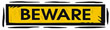Not all trinomials can be factored.

There are trinomials that simply do not comply with these factoring patterns. Not all trinomials can be factored using only integers (or over the set of integers).

Trinomials such as x² + 2x + 7 or 3x² - x - 5 will not play by these factoring rules.
These trinomials, over the set of integers, are called prime polynomials.

In the Quadratics section, we will find additional procedures that will be helpful when attempting to factor polynomials that cannot be factored over the set of integers.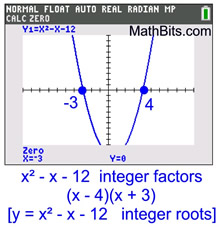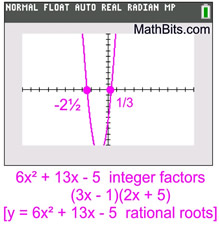These first graphs show functions defined by expressions which are factorable over the set of integers.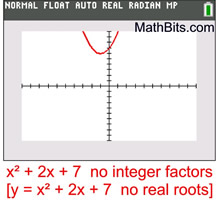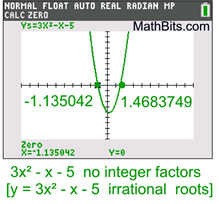These second graphs show functions defined by expressions which are NOT factorable over the set of integers.These are prime polynomials over the set of integers.Factor: x2 + 5x + 6 using Algebra Tiles

KEY: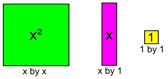See more about Algebra Tiles.
 Place the x2 tile, 5 x-tiles, and 6 1-tiles in the grid. Be sure to keep straight lines inside the grid.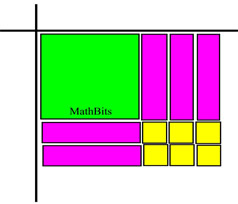Fill the outside sections of the grid with x-tiles and 1-tiles that complete the pattern.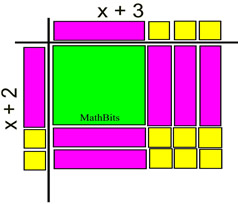The graphing calculator can prove to be helpful for checking your answer when factoring over the set of integers.For help with factoring on your calculator, Click Here!